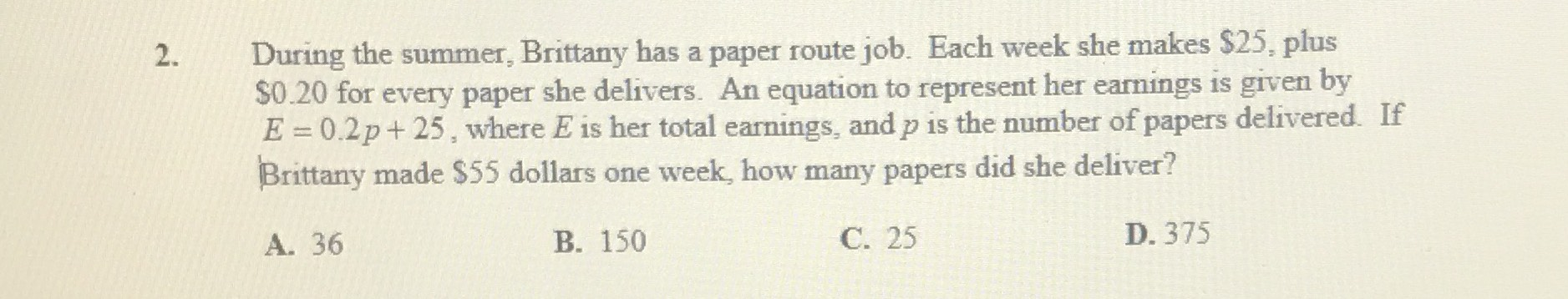### ¿Todavía tienes preguntas de matemáticas?

Pregunte a nuestros tutores expertos
Algebra
Pregunta2. During the summer, Brittany has a paper route job. Each week she makes $$\ 25 ,$$ plus

$$\ 0.20$$ for every paper she delivers. An equation to represent her earnings is given by

$$E = 0.2 p + 25$$ , where $$E$$ is her total earnings, and $$p$$ is the number of papers delivered. If Brittany made $$\ 55$$ dollars one week, how many papers did she deliver?

A. $$36$$ B. $$150$$ C. $$25$$ D. $$375$$